# RD Sharma Class 8 Solutions Chapter 12 Percentage Ex 12.2

In this chapter, we provide RD Sharma Class 8 Solutions Chapter 12 Percentage Ex 12.2 for English medium students, Which will very helpful for every student in their exams. Students can download the latest RD Sharma Class 8 Solutions Chapter 12 Percentage Ex 12.2 Maths pdf, free RD Sharma Class 8 Solutions Chapter 12 Percentage Ex 12.2 book pdf download. Now you will get step by step solution to each question.

### RD Sharma Solutions for Class 8 Chapter 12 Percentage Ex 12.2Download PDF

Question 1.
Find :
(i) 22% of 120
(ii) 25% of Rs. 1000
(iii) 25% of 10 kg
(iv) 16.5% of 5000 metres
(v) 135% of 80 cm
(vi) 2.5% of 10000 ml
Solution: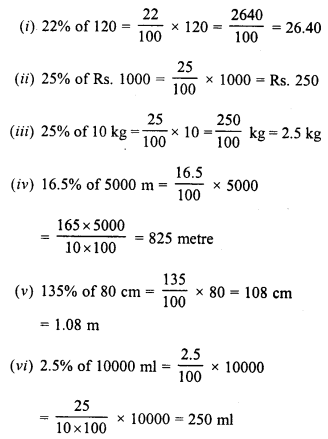Question 2.
Find the number ‘a’, if
(i) 8.4% of a is 42
(ii) 0.5% of a is 3
(iii) 12 % of a is 50
(iv) 100% of a is 100
Solution: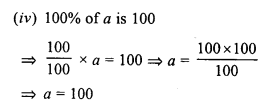Question 3.
x is 5% of y, y is 24% of z. If x = 480, find the values of y and z.
Solution:
x = 5% of y, y = 24% of z.
x = 480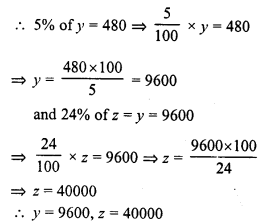Question 4.
A coolie deposits Rs. 150 per month in his post office Saving Bank account. If this is 15% of his monthly income, find his monthly income.
Solution:
Let his monthly income = Rs. x
15% of x = Rs. 150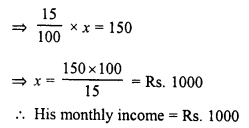Question 5.
Asha got 86.875% marks in the annual examination. If she got 695 marks, find the number of marks of the Examination.
Solution:
Let total marks of the examination = x
86.875% of x = 695
=> 86.875 x 1100 x x = 695Question 6.
Deepti went to school for 216 days in a full year. If her attendance is 90%, find the number of days on which the school was opened ?
Solution:
Let the school opened for = x days = 90% of x = 216Question 7.
A garden has 2000 trees. 12% of these are mango trees, 18% lemon and the rest are orange trees. Find the number of orange trees.
Solution:
Number of total trees = 2000Rest trees = 2000 – (240 + 360) = 2000 – 600 = 1400
Number of orange trees = 1400

Question 8.
Balanced diet should contain 12% of protein, 25% of fats and 63% of carbohydrates. If a child needs 2600 calories in this food daily, find in calories the amount of each of these in his daily food in take.
Solution:
Balance diet contains
Protein = 12%
Fats = 25%
Carbohydrates = 63%
Number of total calories = 2600
Number of calories of proteins = 12% of 2600 = 12100 x 2600 = 312
Number of calories of fats = 25% of 2600 = 25100 x 2600 = 650
Number of calories of carbohydrates = 63% of 2600 = 63100 x 2600 = 1638

Question 9.
A cricketer scored a total of 62 runs in 96 balls. He hits 3 sixes, 8 fours, 2 twos and 8 singles. What percentage of the total runs came in :
(i) Sixes
(ii) Fours
(iii) Twos
(iv) Singles
Solution:
Total score of a cricketer = 62 runs
(z) Number of sixes = 3
Run from 3 sixes = 3 x 6 = 18
Percentage = 1862 x 100 = 29.03%
(ii) Number of fours = 8
Total run from 8 fours = 4 x 8 = 32
Percentage = 3262 x 100 = 51.61%
(iii) Number of twos = 2
Total score from 2 twos = 2 x 2 = 4
Percentage = 462 x 100 = 40062 = 6.45%
(iv) Number of single run = 8
Percentage = 862 x 100 = 80062 = 12.9%

Question 10.
A cricketer hits 120 runs in 150 balls during a test match. 20% of the runs came in 6’s, 30% in 4’s, 25% in 2’s and the rest in 1’s. How many runs did he score in :
(i) 6’s
(ii) 4’s
(iii) 2’s
(iv) singles
What % of his shots were scoring ones ?
Solution:
Total runs scored by a cricketer =120
(i) Number of runs from sixes (6’s) = 20% of 120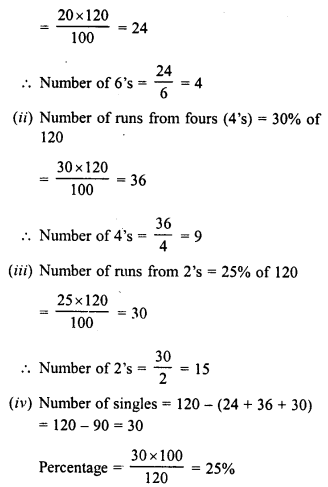Question 11.
Radha earns 22% of her investment. If she earns Rs. 187, then how much did she invest ?
Solution:
Total earning from investment = Rs. 187
Percent earning = 22%
Let his investment = x
Then 22% of x = Rs. 187Question 12.
Rohit deposits 12% his income in a bank. He deposited Rs. 1440 in the bank during 1997. What was his total income for the year 1997 ?
Solution:
Deposit in the bank = Rs. 1440
Percentage = 12% of his total income
Let his total income = Rs. x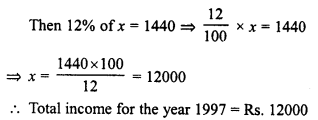Question 13.
Gunpowder contains 75% nitre and 10% sulphur. Find the amount of the gunpowder which carries 9 kg nitre. What amount of gunpowder would contain 2.3 kg sulphur ?
Solution:
(i) In gunpowder,
Nitre = 75%
Sulphur = 10%
Let total amount of gunpowder = x kg
Nitre = 9 kgQuestion 14.
An alloy of tin and copper consists of 15 parts of tin and 105 parts of copper. Find the percentage of copper in the alloy ?
Solution:
In an alloy,
Number of parts of tin = 15
and number of parts of copper = 105
Total parts = 15 + 105 = 120
Percentage of copper in the alloy = 105120 x 100 = 87.5%

Question 15.
An alloy contains 32% copper, 40% nickel and rest zinc. Find the mass of the zinc in 1 kg of the alloy.
Solution:
In an alloy,
Copper = 32%
Nickel = 40%
Rest is zinc = 100 – (32 + 40) = 100 – 72 = 28%
Mass of zinc in 1 kg = 28% of 1 kg = 28100 x 100 gm = 280 gm.

Question 16.
A motorist travelled 122 kilometres before his first stop. If he had 10% of his journey to complete at this point, how long was the total ride ?
Solution:
Distance travelled before first stop = 122 km
Let total journey = x km
10% of x = 122Question 17.
A certain school has 300 students, 142 of whom are boys. It has 30 teachers, 12 of whom are men. What percent of the total number of students and teachers in the school is female ?
Solution:
Total numbers of teachers = 30
Number of male teachers = 12
Number of female teacher = 30 – 12 = 18
Percentage of female teachers = 18×10030 = 60%

Question 18.
Aman’s income is 20% less than that of Anil. How much percent is Anil’s income more than Aman’s income ?
Solution:
Let Anil’s income = Rs. 100
Then Aman’s income = Rs, 100 – 20 = Rs. 80
Now, difference of both’s incomes = 100 – 80 = Rs. 20
Anil income is Rs. 20 more than that of Aman’s
Percentage = 20×10080 = 25%

Question 19.
The value of a machine depreciates every year by 5%. If the present value of the machine be Rs. 100000, what will be its value after 2 years ?
Solution:
Present value of machine = Rs. 100000
Rate of depreciation per year = 5%
Period = 2 years
Value of machine after 2 years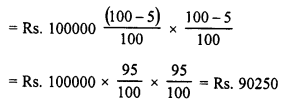Question 20.
The population of a town increases by 10% annually. If the present population is 60000, what will be its population after 2 years ?
Solution:
Present population of the town = 60000
Increase annually = 10%
Period = 2 years
Population after 2 years will beQuestion 21.
The population of a town increases 10% annually. If the present population is 22000, find its population a year ago.
Solution:
Let the population of the town a year ago was = x
Increase in population = 10%Question 22.
Ankit was given an increment of 10% on his salary. His new salary is Rs. 3575. What was his salary before increment ?
Solution:
Let the salary of Ankit before increment = x
Increment given = 10% of the salary
Salary after increment will beQuestion 23.
In the new budget, the price of petrol rose by 10%. By how much percent must one reduce the consumption so that the expenditure does not increase ?
Solution:
Let price of petrol before budged = Rs. 100
Increase = 10%
Price after budget = Rs. 100 + 10 = Rs. 110
Let the consumption of petrol before budget = 100 l
Price pf 100 l = Rs. 110
Now of new price is Rs. 110, consumption = 100 l
are of new price will be 100, thenQuestion 24.
Mohan’s income is Rs. 15500 per month. He saves 11% of his income. If his income increases by 10% then he reduces his saving by 1%, how much does he save now ?
Solution:
Mohan’s income = Rs. 15500We see that the savings is same
There is no change in savings.

Question 25.
Shikha’s income is 60% more than that of Shalu. What percent is Shalu’s income less than Shikha’s ?
Solution:
Let Shalu’s income = Rs. 100
Then Shikha’s income will be = Rs. 100 + 60 = Rs. 160
Now difference in their incomes = Rs. 160 – 100 = Rs. 60
Shalu’s income is less than Shikha’s income by Rs. 60
Percentage less = 60×100160 = 752 % = 37.5%

Question 26.
Rs. 3500 is to be shared among three people so that the first person gets 50% of the second who in turn gets 50% of the third. How much will each of them get ?
Solution:
Let the third person gets = Rs. x
Then second person will getQuestion 27.
After a 20% hike, the cost of Chinese Vase is Rs. 2000. What was the original price of the object ?
Solution:
Let the original price of the vase = Rs. x
Hike in price = 20%Original price of the vase = Rs. 1666.66

All Chapter RD Sharma Solutions For Class 8 Maths

—————————————————————————–

All Subject NCERT Exemplar Problems Solutions For Class 8

All Subject NCERT Solutions For Class 8

*************************************************

I think you got complete solutions for this chapter. If You have any queries regarding this chapter, please comment on the below section our subject teacher will answer you. We tried our best to give complete solutions so you got good marks in your exam.

If these solutions have helped you, you can also share rdsharmasolutions.in to your friends.